# How to Calculate and Solve for the Number of Moles, Volume and Molar Concentration in Chemistry | Nickzom Calculator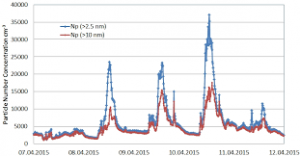The image above represents the molar concentration.

To compute the molar concentration of a substance, two essential parameters are needed and these parameters are number of moles (n) and volume (V).

The formula for calculating the molar concentration:

c = nV

Where;
c = Molar Concentration
n = Number of moles
V = Volume

Let’s solve an example;
Find the molar concentration when the number of moles is 32 with a volume of 120.

This implies that;
n = Number of moles = 32
V = Volume = 120

c = nV
c = 32120
c = 0.266

Therefore, the molar concentration is 0.266 mol/L.

Calculating the Number of Moles when Molar Concentration and Volume is Given.

n = Vc

Where;
n = Number of moles
c = Molar Concentration
V = Volume

Let’s solve an example;
Find the number of moles when the molar concentration is 40 with a volume of 31.

This implies that;
c = molar concentration = 40
V = Volume = 31

n = Vc
n = 31 x 40
n = 1240

Therefore, the Number of Moles is 1240 mol.

Calculating the Volume when Molar Concentration and Number of Moles is Given.

V = n / c

Where;
V = Volume
n = Number of moles
c = Molar Concentration

Let’s solve an example;
Find the volume when the molar concentration is 14 with a number of moles of 44.

This implies that;
c = molar concentration = 14
n = Number of moles = 44

V = n / c
V = 44 / 14
V = 0.318

Therefore, the volume is 0.318.

Nickzom Calculator – The Calculator Encyclopedia is capable of calculating the molar concentration.

To get the answer and workings of the molar concentration using the Nickzom Calculator – The Calculator Encyclopedia. First, you need to obtain the app.

You can get this app via any of these means:

To get access to the professional version via web, you need to register and subscribe for NGN 1,500 per annum to have utter access to all functionalities.
You can also try the demo version via https://www.nickzom.org/calculator

Once, you have obtained the calculator encyclopedia app, proceed to the Calculator Map, then click on Basic Chemistry under Chemistry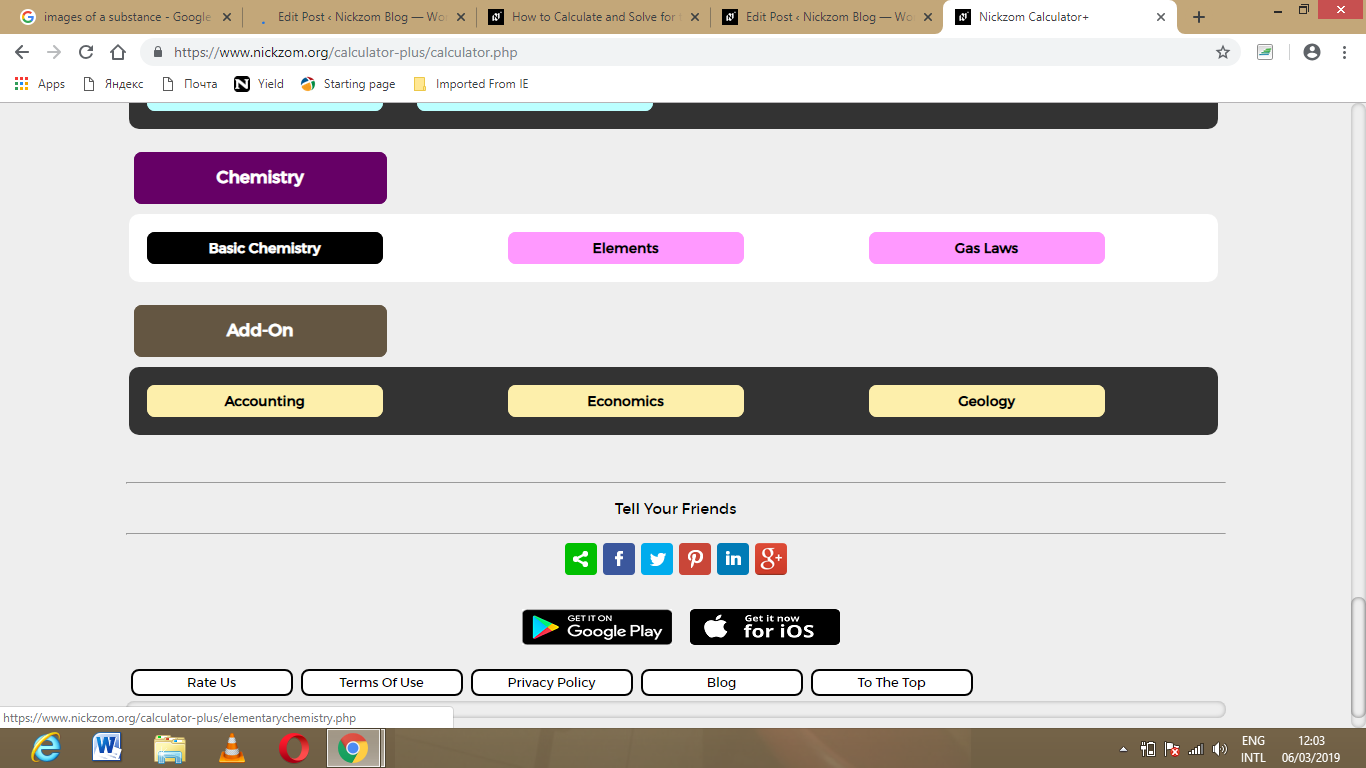Now, Click on Molar Concentration under Basic Chemistry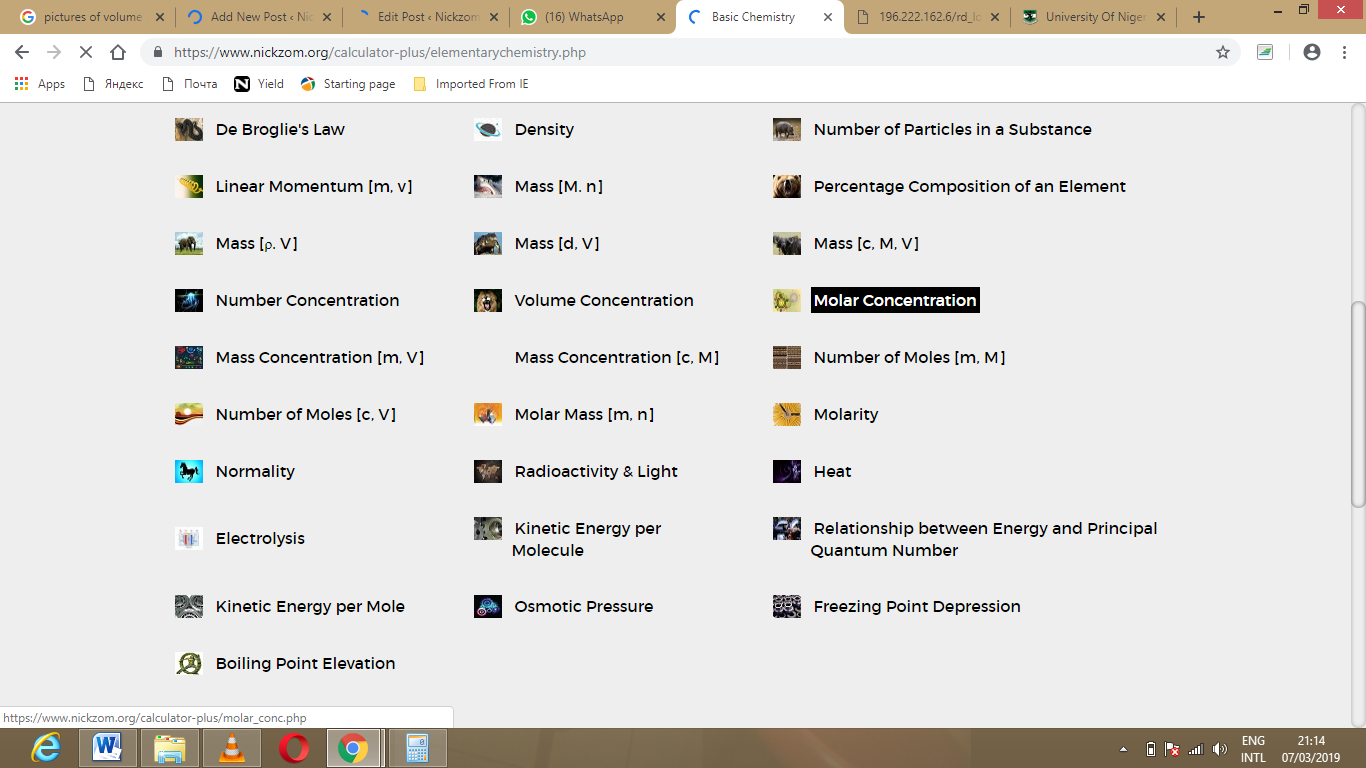The screenshot below displays the page or activity to enter your values, to get the answer for the molar concentration according to the respective parameters which are the number of moles (n) and volume (V).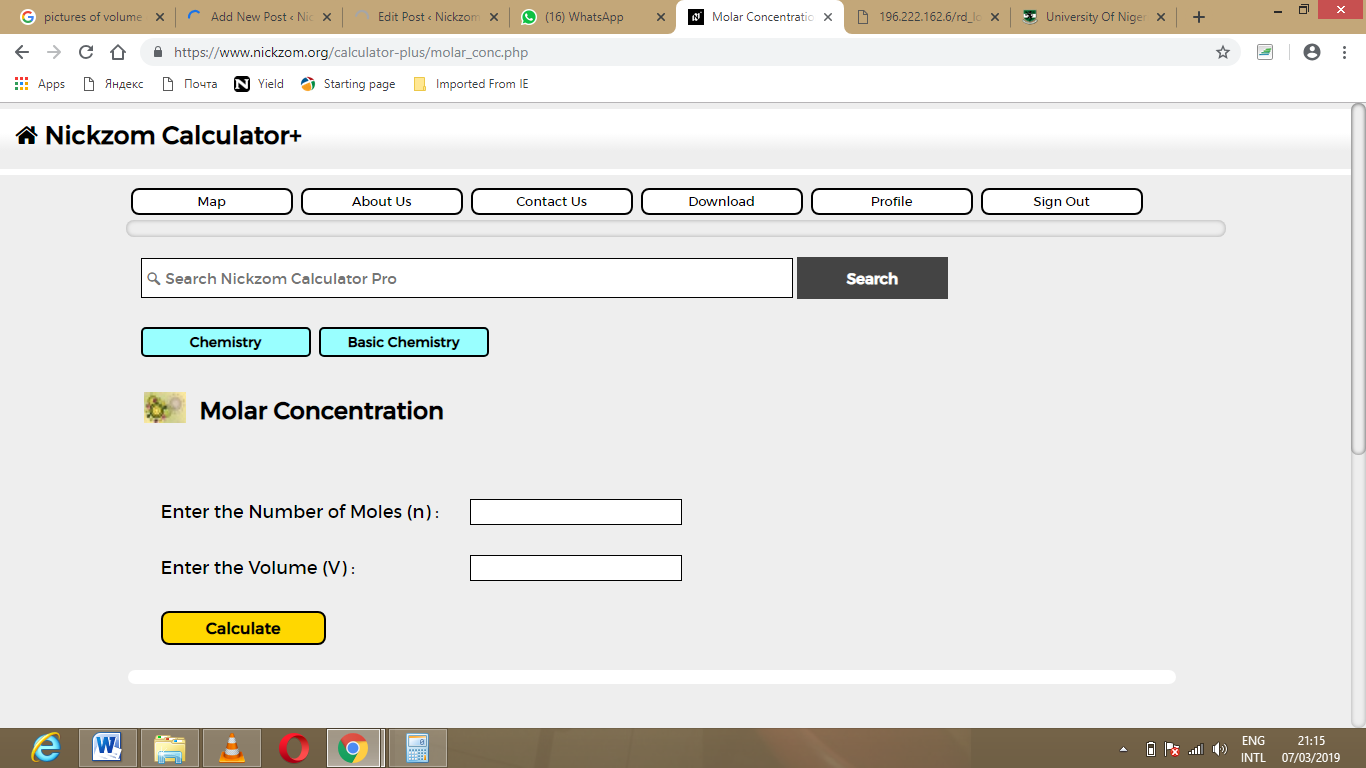Now, enter the values appropriately and accordingly for the parameters as required by the example above where the number of moles (n) is 32 and volume (V) is 120.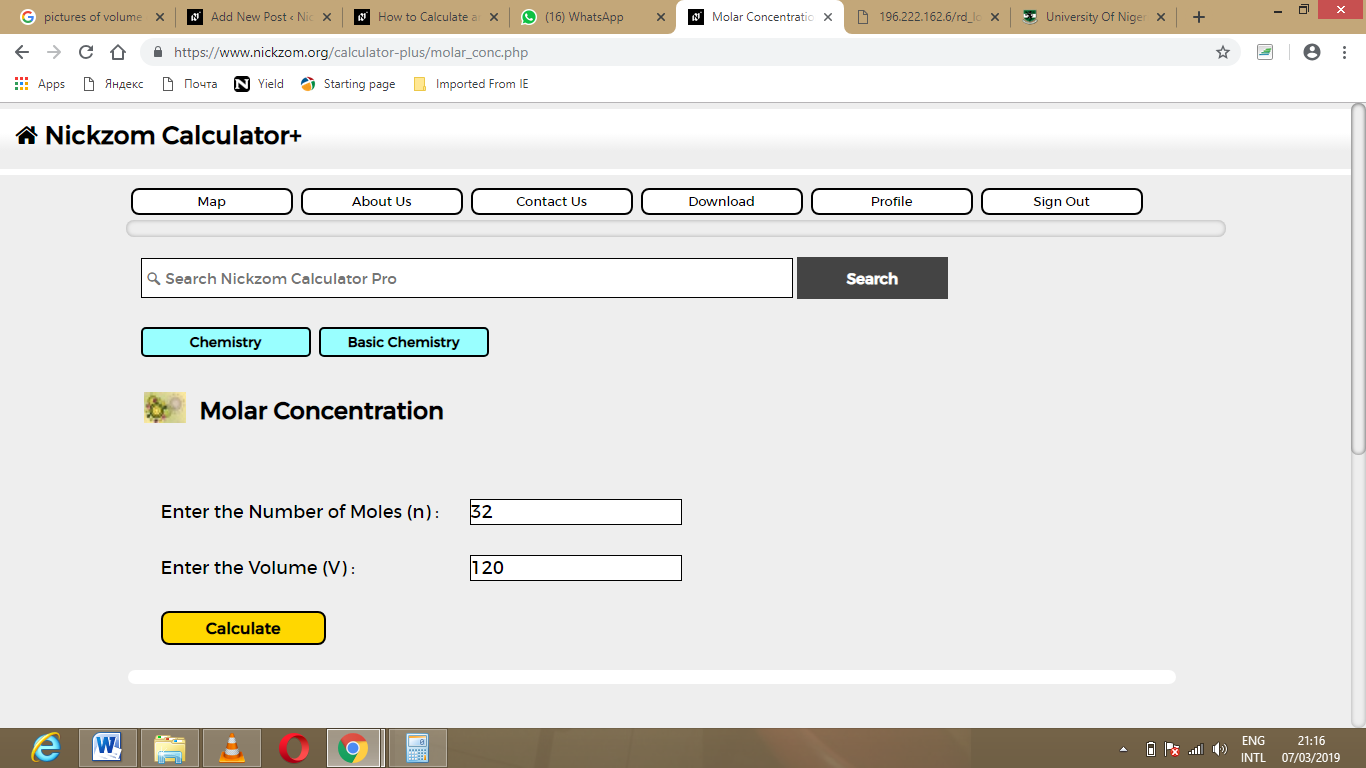Finally, Click on CalculateAs you can see from the screenshot above, Nickzom Calculator – The Calculator Encyclopedia solves for the molar concentration and presents the formula, workings and steps too.